Publicité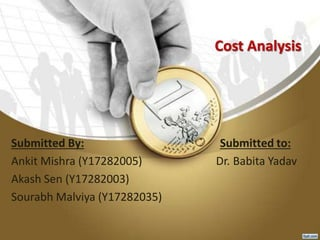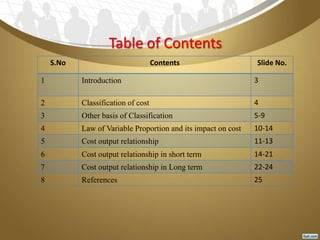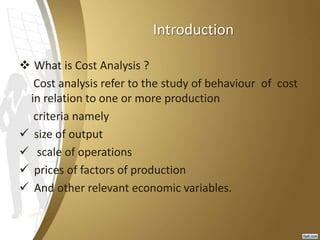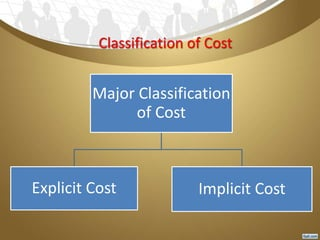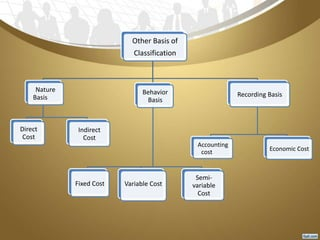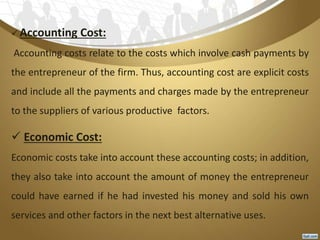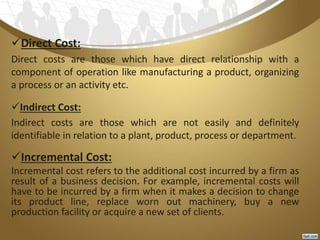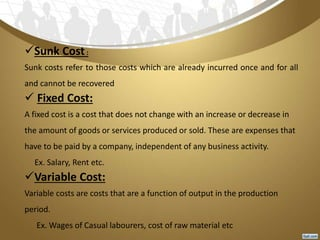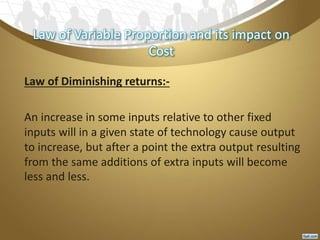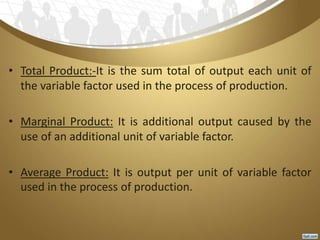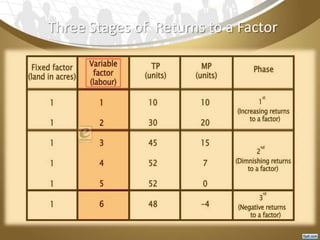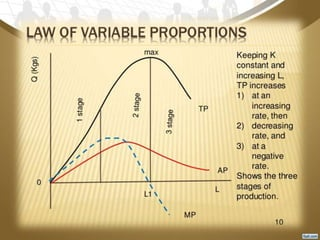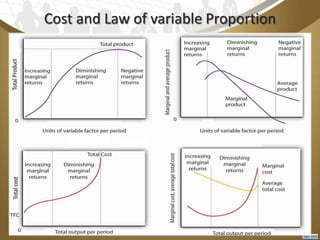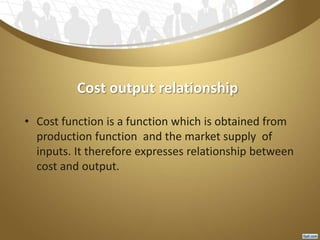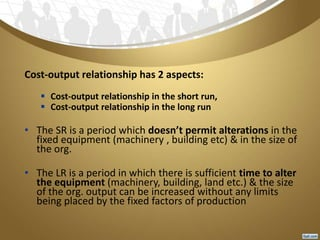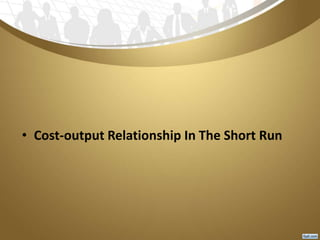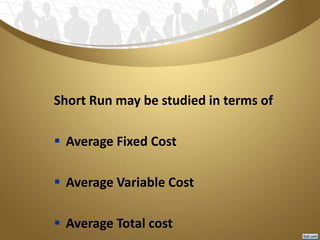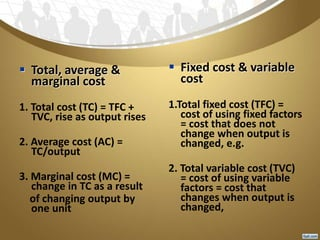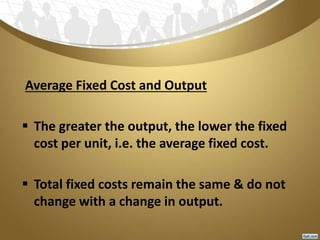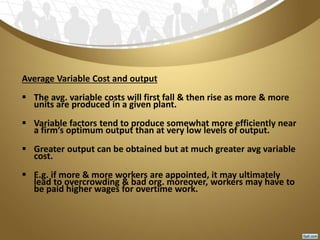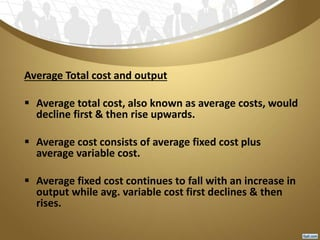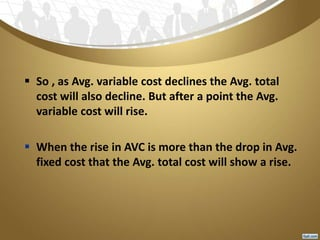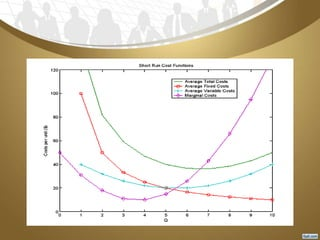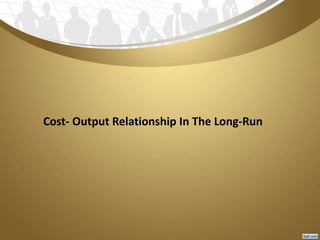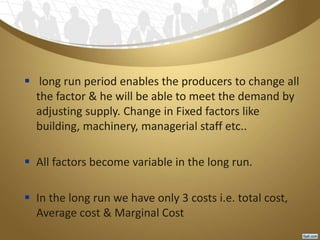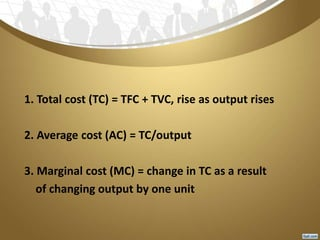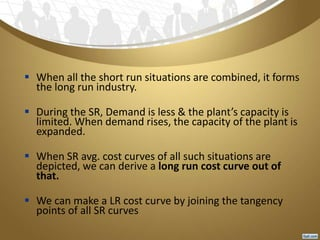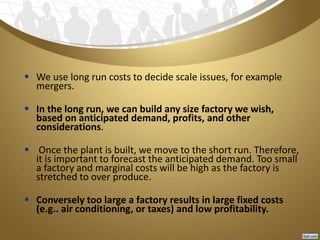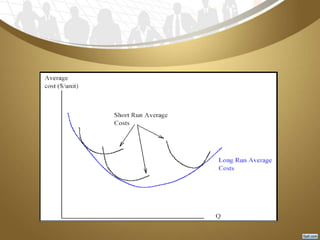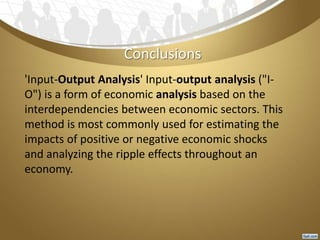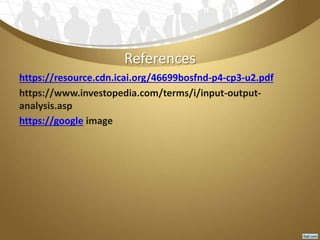1 sur 32
Publicité

### cost analysis

1. Cost Analysis Submitted By: Submitted to: Ankit Mishra (Y17282005) Dr. Babita Yadav Akash Sen (Y17282003) Sourabh Malviya (Y17282035)
2. Table of Contents S.No Contents Slide No. 1 Introduction 3 2 Classification of cost 4 3 Other basis of Classification 5-9 4 Law of Variable Proportion and its impact on cost 10-14 5 Cost output relationship 11-13 6 Cost output relationship in short term 14-21 7 Cost output relationship in Long term 22-24 8 References 25
3. Introduction  What is Cost Analysis ? Cost analysis refer to the study of behaviour of cost in relation to one or more production criteria namely  size of output  scale of operations  prices of factors of production  And other relevant economic variables.
4. Classification of Cost Major Classification of Cost Explicit Cost Implicit Cost
5. What are Explicit and Implicit ? • Explicit Cost : These cost are normally shown in the accounting statement and such arises from transactions between the firm & service of the input or carrying out the production. • Implicit Cost: The cost associated with use of the firm own resources like capital, skills, land etc and therefore such cost is difficult to measure. It is called implicit cost
6. Other Basis of Classification Nature Basis Direct Cost Indirect Cost Behavior Basis Fixed Cost Variable Cost Semi- variable Cost Recording Basis Accounting cost Economic Cost
7.  Accounting Cost: Accounting costs relate to the costs which involve cash payments by the entrepreneur of the firm. Thus, accounting cost are explicit costs and include all the payments and charges made by the entrepreneur to the suppliers of various productive factors.  Economic Cost: Economic costs take into account these accounting costs; in addition, they also take into account the amount of money the entrepreneur could have earned if he had invested his money and sold his own services and other factors in the next best alternative uses.
8. Direct Cost: Direct costs are those which have direct relationship with a component of operation like manufacturing a product, organizing a process or an activity etc. Indirect Cost: Indirect costs are those which are not easily and definitely identifiable in relation to a plant, product, process or department. Incremental Cost: Incremental cost refers to the additional cost incurred by a firm as result of a business decision. For example, incremental costs will have to be incurred by a firm when it makes a decision to change its product line, replace worn out machinery, buy a new production facility or acquire a new set of clients.
9. Sunk Cost: Sunk costs refer to those costs which are already incurred once and for all and cannot be recovered  Fixed Cost: A fixed cost is a cost that does not change with an increase or decrease in the amount of goods or services produced or sold. These are expenses that have to be paid by a company, independent of any business activity. Ex. Salary, Rent etc. Variable Cost: Variable costs are costs that are a function of output in the production period. Ex. Wages of Casual labourers, cost of raw material etc
10. Law of Variable Proportion and its impact on Cost Law of Diminishing returns:- An increase in some inputs relative to other fixed inputs will in a given state of technology cause output to increase, but after a point the extra output resulting from the same additions of extra inputs will become less and less.
11. • Total Product:-It is the sum total of output each unit of the variable factor used in the process of production. • Marginal Product: It is additional output caused by the use of an additional unit of variable factor. • Average Product: It is output per unit of variable factor used in the process of production.
12. Three Stages of Returns to a Factor
13. Cost and Law of variable Proportion
14. Cost output relationship • Cost function is a function which is obtained from production function and the market supply of inputs. It therefore expresses relationship between cost and output.
15. Cost-output relationship has 2 aspects:  Cost-output relationship in the short run,  Cost-output relationship in the long run • The SR is a period which doesn’t permit alterations in the fixed equipment (machinery , building etc) & in the size of the org. • The LR is a period in which there is sufficient time to alter the equipment (machinery, building, land etc.) & the size of the org. output can be increased without any limits being placed by the fixed factors of production
16. • Cost-output Relationship In The Short Run
17. Short Run may be studied in terms of  Average Fixed Cost  Average Variable Cost  Average Total cost
18.  Total, average & marginal cost 1. Total cost (TC) = TFC + TVC, rise as output rises 2. Average cost (AC) = TC/output 3. Marginal cost (MC) = change in TC as a result of changing output by one unit  Fixed cost & variable cost 1.Total fixed cost (TFC) = cost of using fixed factors = cost that does not change when output is changed, e.g. 2. Total variable cost (TVC) = cost of using variable factors = cost that changes when output is changed,
19. Average Fixed Cost and Output  The greater the output, the lower the fixed cost per unit, i.e. the average fixed cost.  Total fixed costs remain the same & do not change with a change in output.
20. Average Variable Cost and output  The avg. variable costs will first fall & then rise as more & more units are produced in a given plant.  Variable factors tend to produce somewhat more efficiently near a firm’s optimum output than at very low levels of output.  Greater output can be obtained but at much greater avg variable cost.  E.g. if more & more workers are appointed, it may ultimately lead to overcrowding & bad org. moreover, workers may have to be paid higher wages for overtime work.
21. Average Total cost and output  Average total cost, also known as average costs, would decline first & then rise upwards.  Average cost consists of average fixed cost plus average variable cost.  Average fixed cost continues to fall with an increase in output while avg. variable cost first declines & then rises.
22.  So , as Avg. variable cost declines the Avg. total cost will also decline. But after a point the Avg. variable cost will rise.  When the rise in AVC is more than the drop in Avg. fixed cost that the Avg. total cost will show a rise.
23. Cost- Output Relationship In The Long-Run
24.  long run period enables the producers to change all the factor & he will be able to meet the demand by adjusting supply. Change in Fixed factors like building, machinery, managerial staff etc..  All factors become variable in the long run.  In the long run we have only 3 costs i.e. total cost, Average cost & Marginal Cost
25. 1. Total cost (TC) = TFC + TVC, rise as output rises 2. Average cost (AC) = TC/output 3. Marginal cost (MC) = change in TC as a result of changing output by one unit
26.  When all the short run situations are combined, it forms the long run industry.  During the SR, Demand is less & the plant’s capacity is limited. When demand rises, the capacity of the plant is expanded.  When SR avg. cost curves of all such situations are depicted, we can derive a long run cost curve out of that.  We can make a LR cost curve by joining the tangency points of all SR curves
27.  We use long run costs to decide scale issues, for example mergers.  In the long run, we can build any size factory we wish, based on anticipated demand, profits, and other considerations.  Once the plant is built, we move to the short run. Therefore, it is important to forecast the anticipated demand. Too small a factory and marginal costs will be high as the factory is stretched to over produce.  Conversely too large a factory results in large fixed costs (e.g.. air conditioning, or taxes) and low profitability.
28. Conclusions 'Input-Output Analysis' Input-output analysis ("I- O") is a form of economic analysis based on the interdependencies between economic sectors. This method is most commonly used for estimating the impacts of positive or negative economic shocks and analyzing the ripple effects throughout an economy.
29. References https://resource.cdn.icai.org/46699bosfnd-p4-cp3-u2.pdf https://www.investopedia.com/terms/i/input-output- analysis.asp https://google image
Publicité